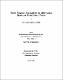## Three magnon excitations in alternating quantum spin/bond chainsIn this thesis we examine the nature of three magnon excitations in alternating spin/bond ferromagnetic chains. We study a Hamiltonian that describes a chain (composed of two non-identical one-dimensional uniform and homogeneous sublattices) with alternating spin magnitudes, S and $S\sp\prime$, and alternating nearest neighbor interactions, $J\sb1$ and $J\sb2.$ The Recursion Method is used to locate the bound states and their relationship to the three-magnon continuum. The specific cases studied are the S = $S\sp\prime$ = 1/2, S = $2S\sp\prime$ = 1, and S = $S\sp\prime$ = 1 alternating spin chains. We consider not only the effects of spin alternation but also of varying strengths. For each case, we studied different bond sets, more exactly, $J\sb1$ = 1 and $J\sb2$ equal to 0, 1/4, 1/2 and 1.